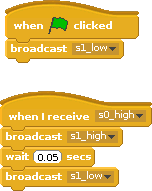# rotary encoder performance

Rotary encoders produce two waveforms, shifted by 90 degrees, which allow to decode position and direction of movements.

The two waveforms look as given in the chart. Only one direction is shown.In raspberry pi forum, someone asked for the performance of a python program to decode these signals.

The code used to validate python program is

```import RPi.GPIO as GPIO
import time

GPIO.setmode (GPIO.BCM)
GPIO.setwarnings(False)
pin_A = 22
pin_B = 27

Encoder_Count = 0      # Encoder Count variable

def do_Encoder(channel):
global Encoder_Count
if GPIO.input(pin_B) == 1:
Encoder_Count += 1
else:
Encoder_Count -= 1

GPIO.setup (pin_A, GPIO.IN, pull_up_down=GPIO.PUD_UP)         # pin input pullup
GPIO.setup (pin_B, GPIO.IN, pull_up_down=GPIO.PUD_UP)         # pin input pullup

GPIO.add_event_detect (pin_A, GPIO.FALLING, callback=do_Encoder)   # Enable interrupt

lastCount = 0
t0 = time.time()
while(1):
t0 += 20
time.sleep(t0 - time.time())
print ("{e:d} diff={d:d}".format(e=Encoder_Count, d = Encoder_Count-lastCount))
lastCount = Encoder_Count

```

As a driver for these signals I use an arduino DUE.

The results for the python code on a Raspberry Pi 3 are

``````freq       exp     measure
10Hz    200         200
100Hz   2000        2000
500Hz  10000       10000  +-2
1000Hz  20000       20000 +-25
2000Hz  40000       40000 -40
3000Hz  60000       60000 -660
5000Hz 100000     -100000 +-20, but received negative values, so python too slow to get matching level``````

Up to 3.000 pulses, the results are quite reliable. For a 1.000RPR encoder, these are 3 rotations per second.

Usually I recommend to use an atmel328-processor as a slave processor to handle time sensitive operations. With an arduino UNO, 16MHz, the results are precise up to 15.000Hz.
There is no complete arduino needed. A bare atmel328, clocked from GPIO4, using level shifters for clock and serial line, would be a cost effective solution.
The sketch uses interrupts for the event inputs:

```const byte ip2 = 2;
const byte ip3 = 3;
volatile long counter = 0;

void ip2Int() {
if ( iip2 == 1 ) {
if ( iip3 == 1 ) {
counter --;
}
else
{
counter ++;
}
}
else // ip2 == 0
{
if ( iip3 == 1 ) {
counter ++;
}
else
{
counter --;
}
}
}

void ip3Int() {
if ( iip2 == 1 ) {
if ( iip3 == 1 ) {
counter ++;
}
else
{
counter --;
}
}
else // ip2 == 0
{
if ( iip3 == 1 ) {
counter --;
}
else
{
counter ++;
}
}
}

void setup() {
Serial.begin(115200);
Serial.println("encoder");

pinMode(ip2, INPUT);
pinMode(ip3, INPUT);

pinMode( 13, OUTPUT);
pinMode(pin_sync, INPUT );

attachInterrupt(digitalPinToInterrupt(ip2), ip2Int, CHANGE);
attachInterrupt(digitalPinToInterrupt(ip3), ip3Int, CHANGE);
}

void loop() {
long c0 = 0;
noInterrupts();
c0 = counter;
interrupts();

Serial.println(c0);
delay(10000);
}```

This code runs up to 15.000Hz, at 20.000 Hz it fails.

An obvious optimization is to change the interrupt routine and avoid the digitalRead-function for the atmel328-processor.

```void ip2Int() {
uint8_t pd = 0b00001100 & PORTD;  // pins 2,3 are PD2, PD3
if ( pd == 0b00001000 || pd == 0b00000100 ) {
counter ++;
}
else {
counter --;
}
}

void ip3Int() {
uint8_t pd = 0b00001100 & PORTD;
if ( pd == 0b00001100 || pd == 0b00000000 ) {
counter --;
}
else {
counter ++;
}
}```

This code is much faster and update rates till 50.000Hz work perfect.

With handcrafted assembler code for the interrupt-routine I would expect to extend performance even more.

Last option investigated was an arduino feather M0 board running at 48MHz.
This produces good results up to 30.000Hz, at 40.000Hz there are failures. With the results from optimized atmel328-code, there is quite a lot of optimization expected.

For the fast running signals, timing accuracy is crucial. To work around this problem, a sync pattern was used: an additional sync signal indicated start and end of measurement from generator to measurement device. Pulse sequence was 10 sec in each case.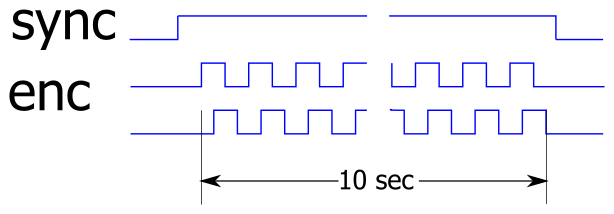For faster signals, either optimized assembler code needs to be used, or a hardware solution with a FPGA.

Updated 2017-02-19: optimized atmel code.

# scratch performance 2, gpioserver reaction time

Perfomance of gpioserver was examined in “gpio performance” ,

Another aspect of gpioserver performance is how fast it can react to gpio pin changes.

The measure response time, I connected an arduino due which raised gpio23 to high and measured time till raspberry responded with a high-value on gpio24.

The arduino code placed a random delay in the start of each measurement cycle in order not to synchronize with scratch. In total more than 10.000 measurements have been taken.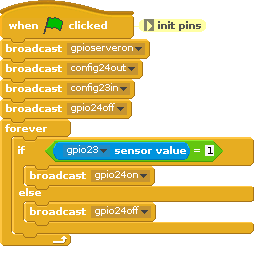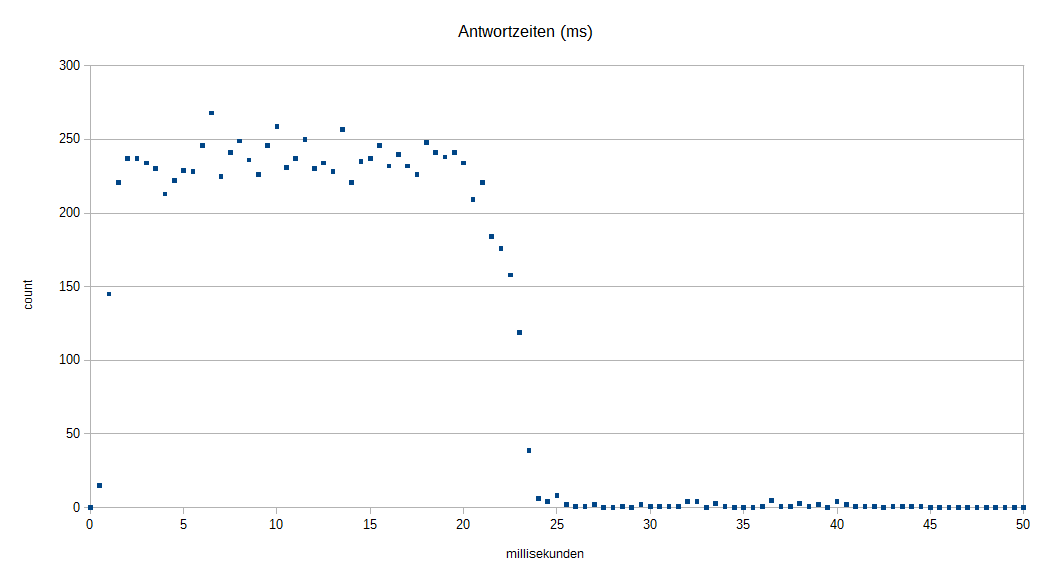The x-axis are the response times. The y-axis are the number of measurements which occurred in this time slot. There is a quite uniform distribution from a few milliseconds up to 24 ms, with a few exceptions up to 40 ms. The measurements are grouped to 0.5ms slots.

Measurement taken on raspberry pi 3, scratch 2016-10-21. The arduino used is a ‘due’, 84MHt and 3.3V which is ideal to interface with raspberry.

# scratch io response times

Scratch 1.4 on raspberry pi allows programs to work with GPIO pins. I was curious to see how fast scratch can react to input values.

To measure these times, I connected an arduino due (84MHz clock) with a raspberry pi 2B. The arduino produces a 0–>1 edge for scratch and scratch responds with a 0–>1-edge on another pin. The red arrow in the chart shows this dependency. The time between these two edges is the response time of scratch.The scratch code is straightforward. Sensor values are 0, 1, so there are logical operations needed.

Response times are aggregated in a chart. X-axes shows the time intervals, y-axis shows how often execution times have been measured in the 0.5 ms intervals.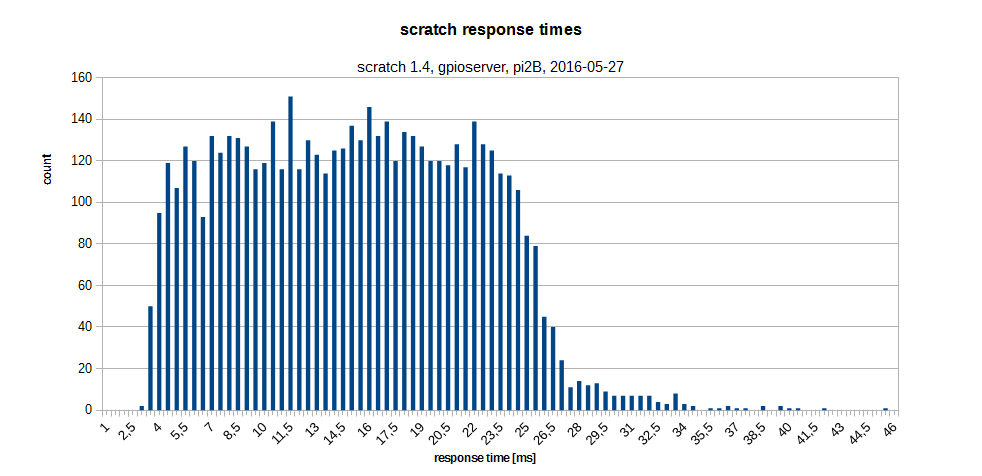The response times range from  3ms to 46ms. The bulk of values is between 5ms and 27ms. The chart is produced from 5659 single measurements. So scratch is sufficient fast to respond in slow environments, but should not be used to control fast systems.

Here the arduino code:

```//
// run on an arduino due

int pout = 11;
int pin = 12;

void setup() {
Serial.begin(9600);
while (!Serial) {
; // wait for serial port to connect. Needed for native USB port only
}
Serial.println("response_time_measurement");
pinMode(pin, INPUT);
pinMode(pout, OUTPUT);
digitalWrite(pout, LOW);
}
long randomnumber = 0;
long t0 = 0;
long t1 = 0;

void loop() {
// wait for input low
while ( HIGH == digitalRead(pin)) ;

randomnumber = random(1, 700);
delay(randomnumber);
t0 = micros();
digitalWrite(pout, HIGH);

while ( LOW == digitalRead(pin)) ;
t1 = micros();

Serial.print(randomnumber);
Serial.print("\t");
Serial.println(t1 - t0);

delay(100);
digitalWrite(pout, LOW);
}
```

There is a variable delay in the arduino code from 1 to 700ms to prevent a ‘lock in’ to scratch cycle times.

Although time measurements are in microseconds, the accuracy depends on how good the compiler is in producing fast code. As the times to be measured are in millisecond range, this approach is reasonable precise.

Similiar setup with scratchClient yields following results: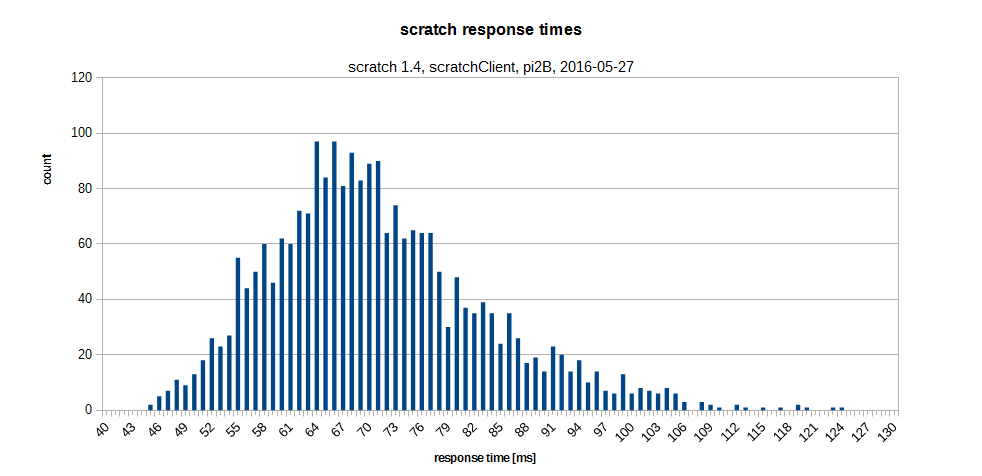Times are centered around some 67 ms, min is 46 ms which is slower than using gpioserver.

The scratchClient-adapter used is ‘GpioButtonInput’, which already provides edge-detection. The scratch code is